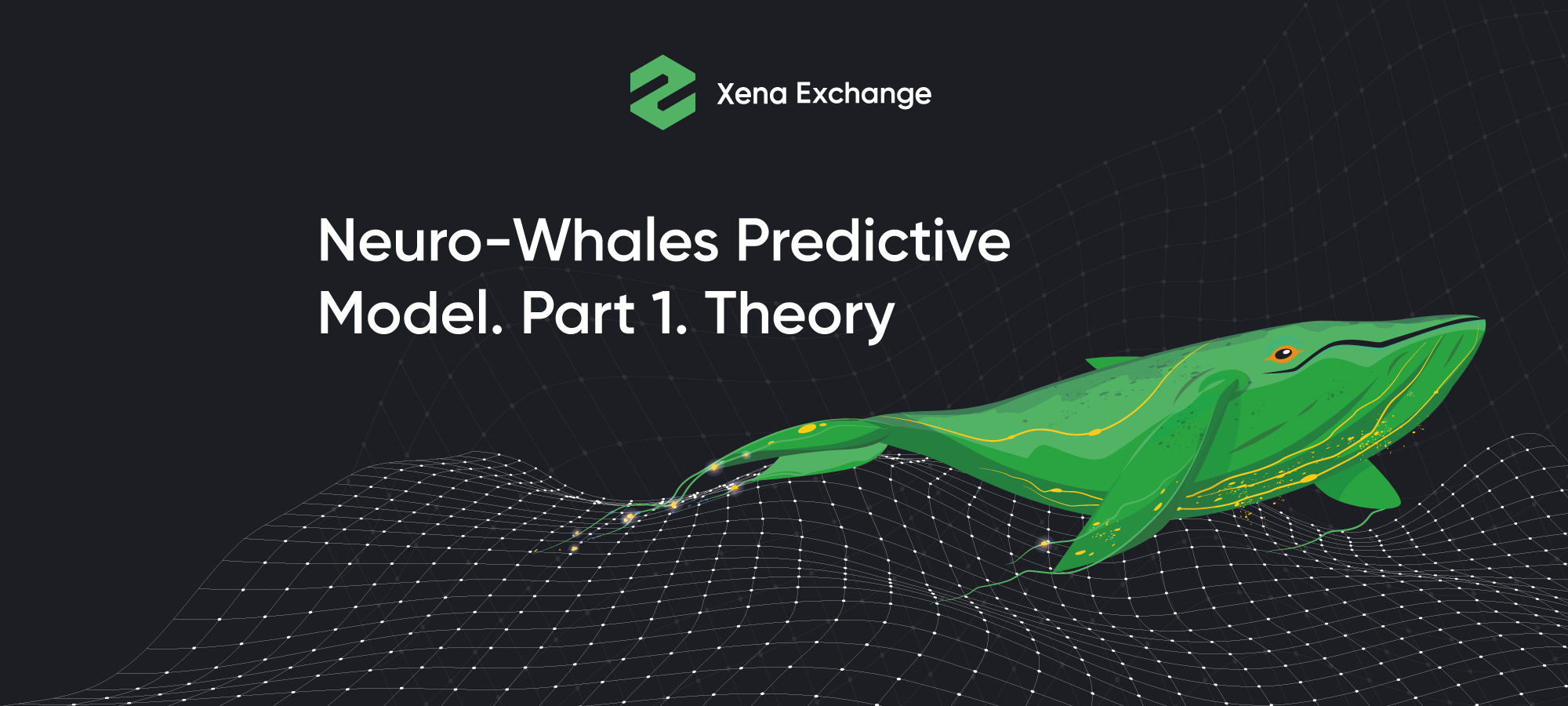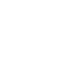# Neuro-Whales Predictive Model. Part 1. TheoryThis series of articles will focus on the intricacies of building neural networks for the purpose of trading in the financial markets. For clarity, let's consider a neural network that I made 2 years ago and refined over the past few months.
The idea for the articles emerged in the course of observations: my quant colleagues and I, delving deeper into the study of the topic of neural networks, more and more often became convinced that the consequences of applications of neural networks are too exaggerated - the labor costs for studying and developing working neural network are much more than, for example, labor costs for writing a pool of strategies. And, if we compare the effectiveness of these two options, then in most cases a portfolio of strategies will be more effective. In practice, only some techniques used in AI (for example, reinforcement learning or genetic algorithms) prove to be effective.
What is a neural network for financial markets?
In its most general form, a neural network for problems of quantitative finance is a black box into which some information is injected:
• what we are trying to predict;
• using which tools we are trying to predict it;
• how we will try to predict it.
At the output we get a prediction of how a certain value will behave in the future on a certain forecast horizon. The amount we are trying to predict will most often be price or volatility. What we will use to predict the value can be roughly divided into two categories:
• derivatives of our value (most often prices)
• out-of-the-market data (tweets, news, statistics)
Let's look at the categories in more detail.
If we use derivative (from the value) instruments, then we mainly deal with indicators. It makes little sense to build a neural network on indicators, since the values ​​of the indicators undergo a number of mathematical transformations during calculations. The more complex the model, the more transformations, which means that the risk of over-optimization becomes the cornerstone of this approach. It turns out that we can optimize indicators and then optimize the neural network on indicators. In this case, we will inevitably get an adjustment - inadequate good results in the test and a complete mismatch of the results when trying to trade it in real time.
What is the reason for this? The reason is the fact that in the process of optimization we will inevitably find the best option, because literally everything will be optimized. In my understanding, optimality cannot exist in this context. A situation that will inevitably happen can be described as follows: imagine that you need to push a bowling ball uphill in such a way that it stops exactly at the top and does not roll away from there. The likelihood that you will succeed is rather small. It is about as small as the likelihood that the market will establish long-term market equilibrium, or that the “optimal F” value will allow you to use trading capital efficiently, or, finally, that the theory of “effective frontier” will allow you to build a stable portfolio of assets.
Let us consider a special case: someone will say that the values ​​of indicators can be , instead of being optimized left as constants, but in this case the meaning of the model is lost, because an indicator is essentially a formula that performs some price transformation, and a neural network is a set of formulas with complex logic and implementation. In this case, over a long period of neural network optimization, it makes no difference whether we feed an indicator or a price as an entry - in any case, we will find a suitable, but probably unstable, result.
So why is it not a good idea to predict a value with derivatives from it? A neural network based on indicators is just a complex and inconvenient implementation of an ordinary trading strategy. This approach has incomparably more risks and disadvantages than the conventional approach to market research and strategy writing.
In the second case, predict the price based on off-market data, we sort of descend to another level of the global market model and look for the root cause of the price movement. The most commonplace example here would be tweets by Elon Musk or D. Trump, which can set the mood for a whole army of investors, induce them to take quick irrational actions and make the price move. Such data are not a consequence or a derivative of the price; on the contrary, they can become good predictors for predicting the direction of prices. With this approach, one can hope that the neural network will show good results.
Let's clarify why we need to make a neural network in the applied sense. The goal of developing any custom indicator or predictor, in my case, is the desire to reduce the time lag between the price update and the indicator / predictor update, or even get ahead of the price value with its predictor. Common indicators from the standard set of any trading terminal (EMA, RSI, Momentum) describe what happened to the price in the past, that is, they essentially describe the past price behavior, transforming it. This means that the standard indicators have a time lag. The value of the time lag depends on the indicator period, in some cases the dependence is nonlinear. In theory, the smaller the time lag, the better the quality (predictive) capabilities of the indicator, so we strive to make a predictor that either reduces the time lag to 0, or makes it negative, that is, it gives us the future price value. This approach is taken from the theory of digital signal processing (if you are interested in this topic, then I will describe it in the next series of articles). Schematically, the result we want to arrive at looks like this:
What neural networks and their parameters are suitable for our main task - price forecasting?
Let's assume that the neural network is still a black box with many toggle switches that we have to design and build. In this case, the first problem that we face is the choice of the shape and appearance of the box.
There are a lot of neural network architectures, but we are interested in recurrent neural networks, since they are well suited for working with time series. We chose recurrent networks for several reasons:
• this is the mainstream for time series forecasting. In my opinion, most scientific works on the use of neural networks in finance use recurrent networks. We will not swim against the tide.
• Empirically researching the topic of applicability of neural networks to trading, I realized for myself that they are the best fit.
The toggle switches on our black box are hyperparameters, when you flip them over, our black box will change its technical properties.
So, the first toggle switch is the activation function. This function converts the input signal to the output using some formula. In our case, we use varieties of exponential functions 'elu', 'selu', 'relu'. The choice of these functions is the result of empirical observations, other functions are also suitable, but these specifically cope with the task at hand. A debatable question, if it is interesting to discuss - write to me (contact details are at the end of the article).
To avoid overadjustment, we will use dropout regularization. This method excludes a certain percentage of random neurons at different iterations (epochs) during training. This will help average the optimization results and not break the model due to unnecessary complexity. In this hyperparameter, we will try dropout with 20% values ​​and will not optimize this value.
Also, the number of neurons in a layer and even the number of layers in the network can be used as hyperparameters. Optimizing the network architecture using these parameters is quite difficult in terms of resources - as the network grows, its calculation will take more and more time, and there will be too many iterations with a full search of hyperparameters.
If you want to go deeper and optimize the entire neural network, then you can additionally manipulate the distribution of the number of neurons over layers using combinatorics. In this case, the number of iterations will grow many times over. We do not need full optimization of the neural network and we will not brute force for all options.
Hint: To efficiently optimize such a neural network, you can use a genetic optimization algorithm.
The neural network has many fine-tune settings. Above, I described a small, but basic part with which to start. Building a good neural network is an art, and anything beyond a neural network textbook or course is a matter of experiment. I doubt that a good neural network can be obtained by a simple enumeration of parameters, therefore, to build a neural network I will use only personal knowledge and accumulated experience, because what is important to us is not an “optimal” model, but one that can be applied in practice.
Attention! It is very important to understand that in terms of practical application we will not build a neural network that will replace the trading algorithm and trade for a whole pool of custom strategies (this is another approach in the field of AI - reinforcement learning). We will make an indicator with a negative time lag, which will allow us to effectively filter trades.
The idea of ​​the model, which will be described in the next part of the article (“Neuro-Whales Predictive Model. Part 2. Practice”) will be quite simple: we will take bitcoin wallets, whose capitalization correlates with the bitcoin price (shifted forward by a certain time), and try to feed their capitalization curves to the input of the neural network. This choice is related to the assumption that there are large players (whales) who know where the bitcoin price will move and buy / sell based on their knowledge. This assumption is based on the asymmetry of the information space around financial markets: big capital accumulates a lot of information.
If you are interested in this topic and want to talk about it with me or discuss your developments, you can write to me directly https://www.linkedin.com/in/hsergeyf/